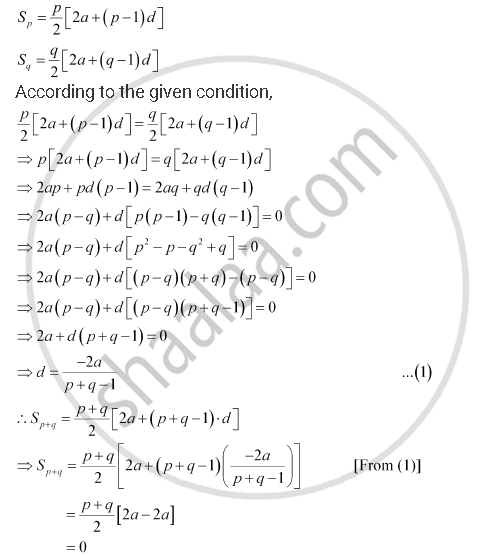CBSE (Arts) Class 11CBSE
Share

# If the Sum of First P Terms of an A.P. is Equal to the Sum of the First Q Terms, Then Find the Sum of the First (P + Q) Terms. - CBSE (Arts) Class 11 - Mathematics

ConceptArithmetic Progression (A.P.)

#### Question

If the sum of first p terms of an A.P. is equal to the sum of the first q terms, then find the sum of the first (p + q) terms.

#### Solution

Let a and d be the first term and the common difference of the A.P. respectively.

Here,Thus, the sum of the first (p + q) terms of the A.P. is 0.

Is there an error in this question or solution?

#### APPEARS IN

NCERT Solution for Mathematics Textbook for Class 11 (2018 to Current)
Chapter 9: Sequences and Series
Q: 10 | Page no. 185

#### Video TutorialsVIEW ALL 

Solution If the Sum of First P Terms of an A.P. is Equal to the Sum of the First Q Terms, Then Find the Sum of the First (P + Q) Terms. Concept: Arithmetic Progression (A.P.).
S